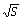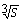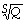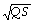/
/
/
20.1   Multiple Choice 1) Assume a stock price of S(0) =
Not my Question
Flag Content

Question : 20.1   Multiple Choice 1) Assume a stock price of S(0) = : 2041225

20.1   Multiple Choice

1) Assume a stock price of S(0) = \$62.00, r = 0.05, σ = 0.30, and dividend = 0. What is the price of a claim that pays? Use formula 20.29.

A) \$7.59

B) \$8.59

C) \$9.59

D) \$10.59

2) Assume a stock price of S(0) = \$83.00, r = 0.045, σ = 0.25, and dividend = 0.02. What is the price of a claim that pays S3? Use formula 20.29.

A) \$423,323

B) \$710,695

C) \$624,165

D) \$818,123

3) Assume a stock price of S(0) = \$45.00, r = 0.03, σ = 0.40, and dividend = 0.015. What is the price of a claim that pays? Use formula 20.29.

A) \$6.41

B) \$5.41

C) \$4.41

D) \$3.41

4) Assume a stock price of S(0) = \$80.00, r = 0.05, σ = 0.35, and dividend = 0.01. What is the price of a claim that pays S-2/3? Use formula 20.29.

A) \$0.25

B) \$0.35

C) \$0.05

D) \$0.15

5) Assume the following: LN(S) and LN(Q) have a correlation coefficient of 0.40, S(0) = 60, Q(0) = 60, r = 0.05, σs = 0.30 σQ = 0.25, and dividend = 0. Using formula 20.39, what is the price of a claim that pays?

A) \$243.96

B) \$322.96

C) \$479.96

D) \$532.96

6) Assume the following: LN(S) and LN(Q) have a correlation coefficient of -0.65, S(0) = 55, Q(0) = 60, r = 0.04, σs = 0.22 σQ = 0.15, and dividends = 0. Using formula 20.39, what is the price of a claim that pays Q/?

A) \$8.16

B) \$9.16

C) \$10.16

D) \$11.16

7) Assume the following: LN(S) and LN(Q) have a correlation coefficient of -0.20, S(0) = 45, S(Q) = 55, r = 0.03, σs = 0.18 σQ = 0.28, and no dividends. Using formula 20.39, what is the price of a claim that pays 1/?

A) \$3.02

B) \$2.02

C) \$1.02

D) \$0.02

8) The value of Z(t) at any point in time can be described as a process in which there is a cumulative effect of infinitely small movements. This process is called:

A) Ornstein-Uhlenbeck

B) Diffusion

C) Ito

D) Geometric

9) A modification to the Brownian process that permits mean reversion is called:

A) Ornstein-Uhlenbeck

B) Diffusion

C) Ito

D) Geometric

10) A modification to the Brownian process in which the drift and volatility depend on the stock price is called:

A) Ornstein-Uhlenbeck

B) Diffusion

C) Ito

D) Geometric

11) For purposes of option pricing, when the movement of a stock price follows a geometric Brownian motion, the stock price is said to follow which type of distribution?

A) Bimodal

B) Latin hypercube

C) Lognormal

D) Normal

12) A Brownian motion is a stochastic process that can be described as a:

A) Pattern of movements with continuous movements

B) Pattern of movements with discrete movements

C) Random walk with continuous movements

D) Random walk with discrete movements

13) The deterministic drift of a pure Brownian motion that is virtually undetectable is sometimes referred to as the:

A) Distribution

B) Expected return

C) Random walk

D) Standard deviation

Solution 5 (1 Ratings )

Solved
Statistics 1 Day Ago 81 Views